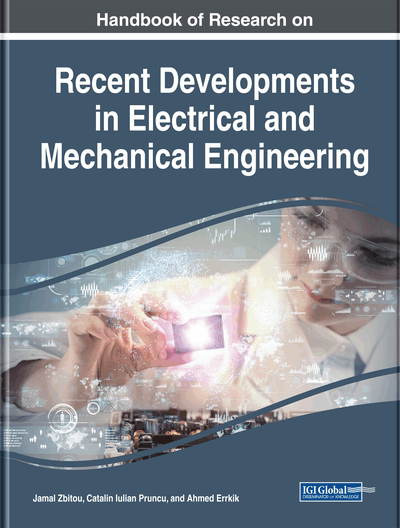# Non-Negative Matrix Factorization for Blind Source Separation

Nabila Aoulass (University Abdelmalek Essaadi, Morocco) and Otman Chakkour (University Abdelmalek Essaadi, Morocco)
DOI: 10.4018/978-1-7998-0117-7.ch009
Available
\$37.50
No Current Special Offers

## Abstract

NMF method aim to factorize a non-negative observation matrix X as the product X =G.F between two non-negative matrices G and F, respectively the matrix of contributions and profiles. Although these approaches are studied with great interest by the scientific community, they often suffer from a lack of robustness with regard to data and initial conditions and can present multiple solutions. The work of this chapter aims to examine the different approaches of NMF, thus introducing the constraint of sparsity in order to avoid local minima. The NMF can be informed by introducing desired constraints on the matrix F (resp G) such as the sum of 1 of each of its lines. Applications on images made it possible to test the interest of many algorithms in terms of precision and speed.
Chapter Preview
Top

## Introduction

Provide The separation of sources is the operation which, from the observations, makes it possible to obtain a set of signals proportional to the sources and to identify the contribution of each of the sources within the observed mixture. Thus, we distinguish two subproblems:

• 1.

the identification of the mixture.

• 2.

the reconstruction of the sources.

This opposite problem is badly posed because without any information on the sources and on the mixture, an infinity of solutions would be admissible. It is then necessary to formulate additional hypotheses and to take into account additional information on mixing and sources. The problem of separation sources can be approached from two points of view. The first is that the decomposition of observations on a basis of elementary signals to eliminate the redundancy of information between the different observations. So, the first methods were proposed by C. Jutten and J. Hérault who realized a nonlinear (ACP) in which we can diagonalize the covariance matrix by the decomposition in eigenvalues (EVD). Due to the limitation of diagonalizable matrices, singular value decomposition (SVD) makes (PCA) always possible based on the orthogonality constraint. It offers the least error (with respect to some measures) with the same reduced complexity, compared to other models. But it is not the only. The NMF is used in place of other low rank factorizations, such as the (SVD) because of its two primary advantages: storage and interpretability. Due to the non-negativity constraints, the NMF produces a so-called “additive parts-based” representation of the data. One consequence of this is that the factors of decomposition matrix are generally naturally sparse, thereby saving a great dea of storage when compared with the (SVD)’s dense factors. But is not for free. On the one hand, the decomposition of the SVD is known to have a polynomial complexity On the other hand, it has been recently demonstrated that the factorization of NMF has a non-deterministic polynomial computation complexity (NP). for which the existence of a optimal algorithm of a polynomial time is unknown. Moreover, non-orthogonal factors do not allow representation as in (PCA) but are used as a basis for unsupervised or prior modeling for supervised learning. A second, more recent approach is that of the Independent Component Analysis (ICA), it will be necessary to wait for the work of P.Comon to generalize this concept. The latter demonstrates, in the case of linear mixtures, that if the source signals are assumed to be mutually independent and non-Gaussian (except for at most one source), it is possible to separate these signals to a scale factor and a permutation by seeking to minimize the dependence measurements between the estimated signals at the output of the separation system. The implicit objective of the (ICA) is often to find physically significant components. However, in some field of environmental science, and using data that has the property of non-negativity, the solutions estimated by the methods based on the (ICA) lack of physical interpretability. In addition, the (ICA) cannot determine the variances (energies) of the independent components as well as the order of the independent sources because the basic functions are classified by non-Gaussianities . In NMF, the non-negativity constraint leads to the representation based on parts of the input mixture that helps to develop structural constraints on the source signals. NMF does not require independent evaluation and is not limited to the length of the data. It provides more important basic vectors for the reconstruction of the underlying signal than the activation vectors. Among the difficulties of matrix factorization in the area of blind separation, the ratio between the number of observations and the number of sources is a problem of interest for a large number of applications and has allowed the taxonomy that we recall below. In most applications and relying on instantaneous linear mixtures, the number m of samples in X is much larger than the numbers n of observations and p of sources. We then separate the determined case p = min (n, m), over-determined p < n, finally underdetermined such that p > min(n,m). When a single-channel source separation problem is considered under-determined, it cannot usually be solved without prior knowledge of the underlying sources in the mixture. For this reason, the problem of estimating multiple overlapping sources from an input mixture is unclear and complex in the (BSS) environment. But (NMF) provides a solution to this single-channel source separation problem by using its non-negativity constraint as well as a supervised mode of operation for source separation.

## Complete Chapter List

Search this Book:
Reset